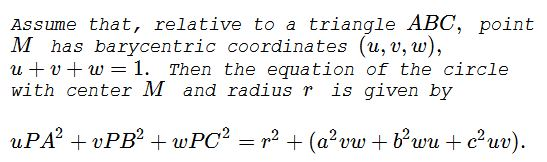# Circles in Barycentric Coordinates

### Problem### Proof

We start with the formula for the distance between two points in barycentric coordinates (Theorem 7, Barycentric Coordinates in Olympiad Geometry, Max Schindler and Evan Chen):

The distance between two points $P(m,n,p)\;$ and $Q(q,s,t),\;$ where the barycentric coordinates relative to $\Delta ABC\;$ satisfy $m+n+p=1\;$ and $q+s+t=1\;$ is defined by

$PQ^2=-a^2(n-s)(p-t)-b^2(p-t)(m-q)-c^2(m-q)(n-s).$

Using that we are able to express distances from $P(x,y,z),\;$ $x+y+z=1\;$ of point $P\;$ on a circle of radius $r\;$ around $M(u,v,w),\;$ $u+v+w=1,\;$ to $A(1,0,0),\;$ $B(0,1,0),\;$ $C(0,0,1),\;$ and $M:$

\begin{align} PA^2&=-a^2yz-b^2z(x-1)-c^2(x-1)y\\ PB^2&=-a^2(y-1)z-b^2zx-c^2x(y-1)\\ PC^2&=-a^2y(z-1)z-b^2(z-1)x-c^2xy\\ PM^2&=-a^2(y-v)(z-w)-b^2(z-w)(x-u)-c^2(x-u)(y-v). \end{align}

Multiply the first of these by $u,\;$ the second by $v,\;$ and the third by $w:$

\begin{align} uPA^2&+vPB^2+wPC^2=\\ &-a^2[(u+v+w)yz-vz-wx]\\ &-b^2[(u+v+w)zx-uz-wx]\\ &-c^2[(u+v+w)xy-uy-vx]\\ &=-a^2[yz-vz-wx]-b^2[zx-uz-wx]-c^2[xy-uy-vx]\\ &=[-a^2(y-v)(z-w)-b^2(z-w)(x-u)-c^2(x-u)(y-v)]\\ &\;\;\;\;+(a^2vw+b^2wu+c^2uv)\\ &=r^2+(a^2vw+b^2wu+c^2uv). \end{align}

### Corollary

The level curves of the function $f(P)=uPA^2+vPB^2+wPC^2\;$ are the circles centered at $u:v:w.$

In particular, for the incenter $I(a:b:c)\;$ of triangle, the values of the function $f(P)=aPA^2+bPB^2+cPC^2\;$ are independent of $P\;$ on circles centered at $I,\;$ which is exactly the previous result concerning the circles concentric with the incircle.

### Acknowledgment

The statement was brought to my attention on google+ by the member under the user name Snail Erato in a comment to my post concerning Another Property of Points on Incircle.

### Applications

The simplest application is to the circles centered at the centroid $G=(1:1:1)=\left(\frac{1}{3},\frac{1}{3},\frac{1}{3}\right).$ If $P\;$ lies on such a circle with radius $r,\;$ then the circle is described by

$\displaystyle \frac{1}{3}(PA^2+PB^2+PC^2)=r^2+\frac{1}{9}(GA^2+GB^2+GC^2).$

This can be rewritten as

$\displaystyle \frac{1}{3}(PA^2+PB^2+PC^2)=r^2+\frac{4}{81}(m_a^2+m_b^2+m_c^2),$

where $m_a,m_b,m_c\;$ are the three medians in $\Delta ABC.\;$ However, say, $\displaystyle m_a^2=\frac{-a^2+2b^2+2c^2}{4},\;$ from which we get

$\displaystyle m_a^2+m_b^2+m_c^2=\frac{3}{4}(a^2+b^2+c^2).$

Such that

$\displaystyle PA^2+PB^2+PC^2=3r^2+\frac{1}{9}(a^2+b^2+c^2),$